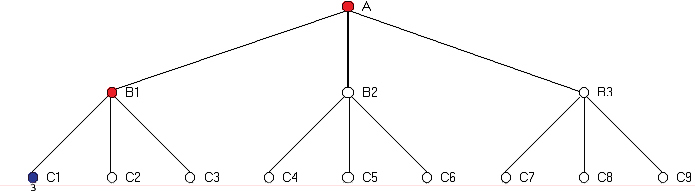ALGORITHMS - ALPHA-BETA PRUNING

Alpha-beta pruning is an improvement over the minimax algorithm. The problem with minimax is that the number of game states it has to examine is exponential in the number of moves. While it is impossible to eliminate the exponent completely, we are able to cut it in half. It is possible to compute the correct minimax decision without looking at every node in the tree. Borrowing the idea of pruning, or eliminating possibilities from consideration without having to examine them, the algorthm allows us to discard large parts of the tree from consideration. When applied to a standard minimax tree, it returns the same move as minimax would, but prunes away branches that cannot possibly influence the final decision.

Alpha-beta pruning can be applied to trees of any depth and it often allows to prune away entire subtrees rather than just leaves. Here is the general algorithm:

1. Consider a node n somewhere in the tree, such that one can move to that node.
2. If there is a better choice m either at the parent of the node n or at any choice point further up, n will never be reached.
3. Once we have enough information about n to reach this conclusion, we can prune it.

Alpha-Beta pruning gets its name from the following parameters:

a = the value of the best choice we have found so far at any choice point along the path for MAX.
ß = the value of the best choice we have found so far at any choice point along the path for MINFig. 1

As shown in Fig. 1, Alpha-Beta search updates the values of a and ß as it goes along and prunes the remaining branches at a node as soon as the value of the current node is known to be worse than the current values of a and ß. The success of the algorithm depends on the order in which the successors are examined.

Transportation tables have been used as a way to keep track of the information about individual nodes. Using such a table can have a framatic effect, sometimes even doubling the reachable search depth. However, if a million nodes are evaluated every second, keeping all the information is a table is not practical.